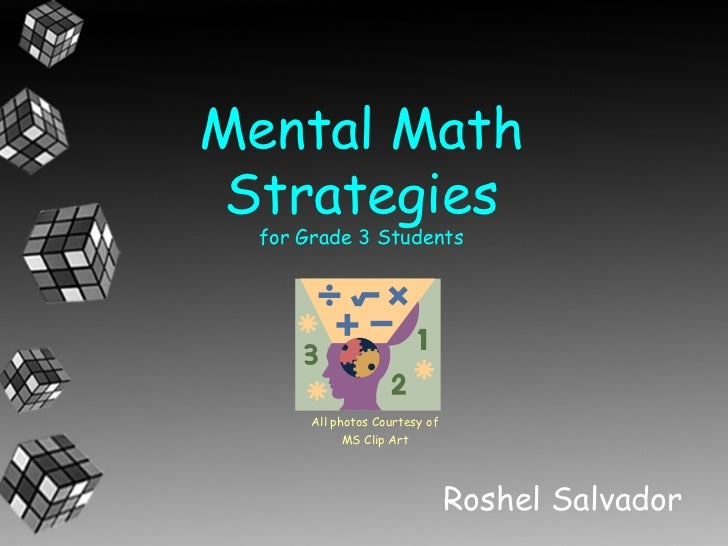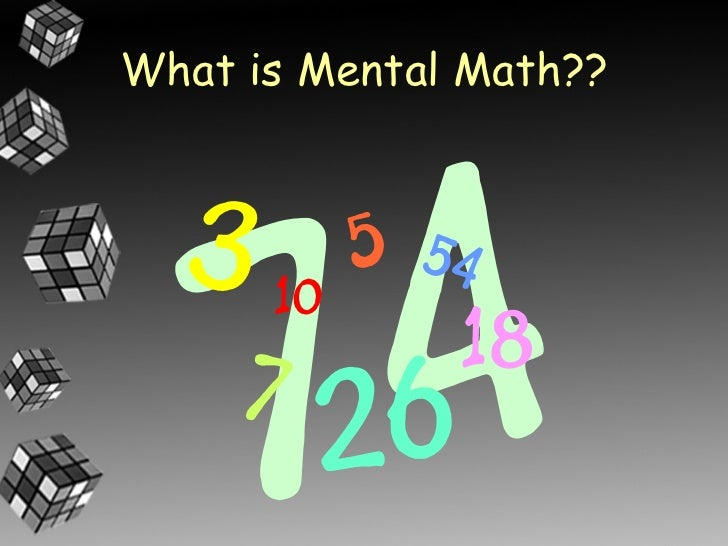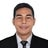Successfully reported this slideshow.You’ve finished this document.
Upcoming SlideShare
EDUKASYON SA PAGPAPAKATAO 2 l7
Next

ofUpcoming SlideShare
EDUKASYON SA PAGPAPAKATAO 2 l7
Next

Share

# Mental Math Strategies for Grade 3

I describe 5 Mental Math Strategies that students can use to help solve math equations.

See all

See all

### Mental Math Strategies for Grade 3

1. 1. Mental Math Strategies for Grade 3 Students All photos Courtesy of MS Clip Art Roshel Salvador
2. 2. What is Mental Math??
3. 3. It’s the ability to solve math equations in your head….
4. 4. WITHOUT usingpaper OR a pencil!
5. 5. With Mental Math you can manipulate (change) your number to make an easier equation. What’s an equation???It’s the number sentence.
6. 6. Here are a few Mental Math strategies: • Count on • Doubles • Near Doubles • Make a Friendly Number • Front-End Adding or Expanded Notation
7. 7. Count On: If one of the numbers being added is a small number, then just count on.
8. 8. Count On: 7+3= 3 is a small number, so just count on to get your answer.
9. 9. Count On: RULE: Usually, if one of the numbers are from 1 to 5, you can just count on.
11. 11. Doubles: Doubles are when you take a number and add it to itself. Eg. 2+2= 9+9= 15+15= 25+25= You double the number.
12. 12. Near Doubles: Knowing your doubles helps with the Near Doubles strategy
13. 13. Near Doubles: Near Doubles almost looks like a double, but it is off by 1,2,3 or 4 Near Double 2 + 2+1 Doubles Doubles
14. 14. Near Doubles:Examples of other near doubles: 11+13= 5+8= 11 + 11+2= 5 + 5+3
15. 15. Near Doubles: You can even use subtraction for near doubles. 11+13= 5+8= 13-2 + 13 8-3 + 8 8+8 - 3 13+13 - 2
16. 16. Make a Friendly Number: What is a friendly number???
17. 17. Make a Friendly Number: A friendly number is a number that ends with a “0”. Numbers with a “0” in the ones spot are easier to add with
18. 18. Make a Friendly Number: For example, to make 28 a friendly number, I can add 2 to it to make it 30
19. 19. Make a Friendly Number:More examples:• Add 5 to 35, to make 40• Subtract 2 from 22 to make 20
20. 20. Make a Friendly Number: This is how it helps: 9 + 4= If I make 9 into a 10, 10 + 3= it is easier to solve because I’m working with a friendly number.
21. 21. Make a Friendly Number: BUT…. Notice how I changed the equation to 10 + 3, NOT 10 + 4 I had to manipulate the equation.Otherwise, I’m just make a new and different equation that won’t give the same answer
22. 22. Make a Friendly Number:In adding, it is called “give and take”.If you give to one side, you have to take from the other side. 9 + 4= 13 (give 1) +1 -1 (take 1) 10 + 3= 13 New equation
23. 23. Make a Friendly Number: In subtracting, it is called “give/give” or “take/take”. If you give to one side, you have to give to the other side. If you take from one side, you have to take from the other side.
24. 24. Make a Friendly Number:Some examples of “give/give” and “take/take”: 9 – 4= 5 (give 1) +1 +1 (give 1) 10 – 5= 5 New equation 11 – 4= 7(take 1) -1 -1 (take 1) 10 – 3= 7 New equation
25. 25. Make a Friendly Number: When you: • “give & take” adding • “give/give”  subtracting • “take/take”  subtracting You are compensating!
27. 27. Front-End AddingExpanded Notation is breaking your number into ones, tens and hundredsEg. 58 42 5 tens, 8 ones 4 tens, 2 ones 50 + 8 40 + 2
28. 28. Front-End Adding• Front-end adding is when you start adding from the left and move to the right Eg. 34 + 45 - start with the tens: 30 + 40 = 70 - now the ones: 4 + 5 = 9 Now you`re working with a friendly number 70 + 9 = 79
29. 29. Let’s Review the Mental Math Strategies • Count on • Doubles • Near Doubles • Make a Friendly Number • Front-End Adding or Expanded Notation
•#### AnnoshKhalil

Sep. 16, 2021
•Jan. 4, 2021
•#### FarhanaEbrahim

Oct. 16, 2020
•#### ManasaSGowda

Sep. 22, 2020
•Sep. 22, 2020
•#### cherrydomingo794

Sep. 10, 2020
•#### poonambatra9

Mar. 29, 2020
•Mar. 18, 2020
•#### TraciCollinsworth

Sep. 11, 2019
•#### BanoKainaat

Jul. 27, 2019
•#### GeetikaGupta52

Feb. 27, 2019
•#### cburt20

Feb. 14, 2019
•#### yanibotto

Sep. 14, 2018
•#### Patishka

Aug. 15, 2018
•#### GRaceBonilla2

Jan. 21, 2018
•#### LuzMoscoso2

Jan. 5, 2018
•#### saranassar5

Dec. 14, 2017
•#### TamikoSachi

Dec. 12, 2017
•Nov. 19, 2017
•#### dianacurammeng

Oct. 15, 2017

Total views

59,093

On Slideshare

0

From embeds

0

Number of embeds

2,052

2,156

Shares

0